# Maharashtra Board Class 11 Physics Solutions Chapter 7 Thermal Properties of Matter

Balbharti Maharashtra State Board 11th Physics Textbook Solutions Chapter 7 Thermal Properties of Matter Textbook Exercise Questions and Answers.

## Maharashtra State Board 11th Physics Solutions Chapter 7 Thermal Properties of Matter

1. Choose the correct option.

Question 1.
The range of temperature in a clinical thermometer, which measures the temperature of the human body, is
(A) 70 ºC to 100 ºC
(B) 34 ºC to 42 ºC
(C) 0 ºF to 100 ºF
(D) 34 ºF to 80 ºF
(B) 34 ºC to 42 ºC

Question 2.
A glass bottle completely filled with water is kept in the freezer. Why does it crack?
(A) Bottle gets contracted
(B) Bottle is expanded
(C) Water expands on freezing
(D) Water contracts on freezing
(C) Water expands on freezingQuestion 3.
If two temperatures differ by 25 °C on Celsius scale, the difference in temperature on Fahrenheit scale is
(A) 65°
(B) 45°
(C) 38°
(D) 25°
(B) 45°

Question 4.
If α, β and γ are coefficients of linear, area l and volume expansion of a solid then
(A) α: β:γ 1:3:2
(B) α:β:γ 1:2:3
(C) α:β:γ 2:3:1
(D) α:β:γ 3:1:2
(B) α:β:γ 1:2:3

Question 5.
Consider the following statements-
(I) The coefficient of linear expansion has dimension K-1
(II) The coefficient of volume expansion has dimension K-1
(A) I and II are both correct
(B) I is correct but II is wrong
(C) II is correct but I is wrong
(D) I and II are both wrong
(A) I and II are both correct

Question 6.
Water falls from a height of 200 m. What is the difference in temperature between the water at the top and bottom of a water fall given that specific heat of water is 4200 J kg-1 °C-1?
(A) 0.96 °C
(B) 1.02 °C
(C) 0.46 °C
(D) 1.16 °C
(C) 0.46 °C

Question 1.
Clearly state the difference between heat and temperature?

 Heat Temperature i. Heat is energy in transit. When two bodies at different temperatures are brought in contact, they exchange heat. OR Heat is the form of energy transferred between two (or more) systems or a system and its surroundings by virtue of their temperature difference. Temperature is a physical quantity that defines the thermodynamic state of a system. OR Heat transfer takes place between the body and the surrounding medium until the body and the surrounding medium are at the same temperature. ii. Heat exchange can be measured with the help of a calorimeter. Temperature is measured with the help of a thermometer. iii. Heat (being a form of energy) is a derived quantity. Temperature is a fundamental quantity.

Question 2.
How a thermometer is calibrated?

1. For the calibration of a thermometer, a standard temperature interval is selected between two easily reproducible fixed temperatures.
2. The fact that substances change state from solid to liquid to gas at fixed temperatures is used to define reference temperature called fixed point.
3. The two fixed temperatures selected for this purpose are the melting point of ice or freezing point of water and the boiling point of water.
4. This standard temperature interval is divided into sub-intervals by utilizing some physical property that changes with temperature.
5. Each sub-interval is called as a degree of temperature. Thus, an empirical scale for temperature is set up.Question 3.
What are different scales of temperature? What is the relation between them?

1. Celsius scale:
• The ice point (melting point of pure ice) is marked as O °C (lower point) and steam point (boiling point of water) is marked as 100 °C (higher point).
• Both are taken at one atmospheric pressure.
• The interval between these points is divided into two equal parts. Each of these parts is called as one degree celsius and it is ‘written as 1 °C.
2. Fahrenheit scale:
• The ice point (melting point of pure ice) is marked as 32 °F and steam point (boiling point of water) is marked as 212 °F.
• The interval between these two reference points is divided into 180 equal parts. Each part is called as degree fahrenheit and is written as 1 °F.
3. Kelvin scale:
• The temperature scale that has its zero at -273.15 °C and temperature intervals are same as that on the Celsius scale is called as kelvin scale or absolute scale.
• The absolute temperature, T and celsius temperature, TC are related as, T = TC + 273.15
eg.: when TC = 27 °C,
T = 27+273.15 K = 300.15 K

Relation between different scales of temperature:
$$\frac{\mathrm{T}_{\mathrm{F}}-32}{180}=\frac{\mathrm{T}_{\mathrm{C}}-0}{100}=\frac{\mathrm{T}_{\mathrm{K}}-273.15}{100}$$
where,
TF = temperature in fahrenheit scale,
TC = temperature in celsius scale,
TK = temperature in kelvin scale,
[Note: At zero of the kelvin scale, every substance in nature has the least possible activity.]

Question 4.
What is absolute zero?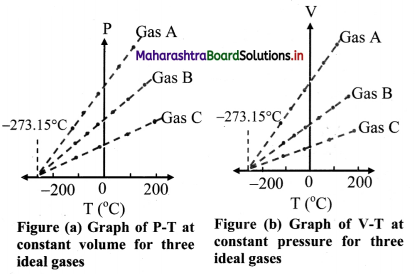1. When the graph of pressure (P) against temperature T (°C) at constant volume for three ideal gases A, B and C is plotted, in each case, P -T graph is straight line indicating direct proportion between them. The slopes of these graphs are different.
2. The individual straight lines intersect the pressure axis at different values of pressure at O °C. but each line intersects the temperature axis at the same point, i.e., at absolute temperature (-273.15 °C).
3. Similarly graph at constant pressure for three different ideal gases A, B and C extrapolate to the same temperature intercept -273.15 °C i.e., absolute zero temperature.
4. It is seen that all the lines for different gases Cut the temperature axis at the same point at -273.15 °C.
5. This point is termed as the absolute zero of temperature.
6. It is not possible to attain a temperature lower than this value. Even to achieve absolute zero temperature is not possible in practice.
[Note: The point of zero pressure or zero volume does not depend on am specific gas.]

Question 5.
Derive the relation between three coefficients of thermal expansion.
Consider a square plate of side l0 at 0 °C and h at T °C.

1. lT = l0 (1 + αT)
If area of plate at 0 °C is A0, A0 = $$l_{0}^{2}$$
If area of plate at T °C is AT,
AT = $$l_{\mathrm{T}}^{2}=l_{0}^{2}$$ (1 + αT)2
or AT = A0 (1 + αT)2 …………… (1)
Also,
AT = A0(1 + βT)2 …………… (2)
……………. [∵ β = $$\frac{\mathrm{A}_{\mathrm{T}}-\mathrm{A}_{0}}{\mathrm{~A}_{0}\left(\mathrm{~T}-\mathrm{T}_{0}\right)}$$]
2. Using Equations (1) and (2),
A0 (1 + αT)2 = A0(1 + βT)
∴ 1 + 2αT + α2T2 = 1 + βT
3. Since the values of a are very small, the term α2T2 is very small and may be neglected,
∴ β = 2a
4. The result is general because any solid can be regarded as a collection of small squares.

Relation between coefficient of linear expansion (α) and coefficient of cubical expansion (γ).

1. Consider a cube of side l0 at 0 °C and lT at T °C.
∴ lT = l0(1 + αT)
If volume of the cube at 0 °C is V0, V0 = $$l_{0}^{3}$$
If volume of the cube at T °C is
VT, VT = $$l_{\mathrm{T}}^{3}=l_{0}^{3}$$ (1 + αT)3
VT = V0 (1 + αT)3 ………. (1)
Also,
VT = V0(1 + γT) …………. (2)
…………. [∵ γ = $$\frac{\mathrm{V}_{\mathrm{T}}-\mathrm{V}_{0}}{\mathrm{~V}_{0}\left(\mathrm{~T}-\mathrm{T}_{0}\right)}$$]
2. Using Equations (1) and (2),
V0(1 + αT)3 = V0(1 + γT)
∴ 1 + 3αT + 3α2T2 + α3T3 = 1 + γT
3. Since the values of a are very small, the terms with higher powers of a may be neglected,
∴ γ = 3α
4. The result is general because any solid can be regarded as a collection of small cubes.

Relation between α, β and γ is given by,
α = $$\frac{\beta}{2}=\frac{\gamma}{3}$$
where, α = coefficient of linear expansion.
β = coefficient of superficial expansion,
γ = coefficient of cubical expansion.Question 6.
State applications of thermal expansion.
Applications of thermal expansion:

• The steel wheel is heated to expand. This expanded wheel can easily fit over axle. The wheel is then cooled quickly. Upon cooling, wheel contracts and fits tightly upon the axle.
• An electric light bulb gets hot quickly when in use. The wire leads to the filament are sealed into the glass. 1f the glass of the bulbs has significantly different thermal expansivity from the wire leads, the glass and the wire would separate, breaking down the vacuum. To prevent this, wires are made of platinum or some suitable alloy with the same expansivity as ordinary glass.

Question 7.
Why do we generally consider two specific heats for a gas?

• A slight change in temperature causes considerable change in pressure as well as volume of the gas.
• Therefore, two principal specific heats are defined for a gas viz., specific heat capacity at constant volume (SV) and specific heat capacity at constant pressure (Sp).

Question 8.
Are freezing point and melting point same with respect to change of state ? Comment.
Though freezing point and melting point mark same temperature (0°C or 32° F), state of change is different for the two points. At freezing point liquid gets converted into solid, whereas at melting point solid gets converted into its liquid state.Question 9.
Define
(i) Sublimation
(ii) Triple point.

1. The change from solid state to vapour stale without passing through the liquid state is called sublimation and the substance is said to sublime.
Examples: Dry ice (solid CO2) and iodine.
2. The triple point of water is that point where water in a solid, liquid and gas state co-exists in equilibrium and this occurs only at a unique temperature and a pressure.

Question 10.

1. When one end of a metal rod is heated, the heat flows by conduction from hot end to the cold end.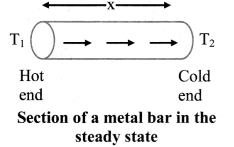2. As a result, the temperature of every section of the rod starts increasing.
3. Under this condition, the rod is said to be in a variable temperature state.
4. After some time, the temperature at each section of the rod becomes steady i.e., does not change.
5. Temperature of each cross-section of the rod now becomes constant though not the same. This is called steady state condition.

Question 11.
Define coefficient of thermal conductivity. Derive its expression.
Coefficient of thermal conductivity of a material is defined as the quantity of heat that flows in one second between the opposite faces of a cube of side 1 m, the faces being kept at a temperature difference of 1°C (or 1 K).

Expression for coefficient of thermal conductivity:

1. Under steady state condition, the quantity of heat ‘Q’ that flows from the hot face at temperature T1 to the cold face at temperature T2 of a cube with side x and area of cross-section A is
• directly proportional to the cross-sectional area A of the face. i.e.. Q ∝ A
• directly proportional to the temperature difference between the two faces i.e., Q ∝ (T1 – T2)
• directly proportional to time t (in seconds) for which heat flows i.e.. Q ∝ t
• inversely proportional to the perpendicular distance x between hot and cold faces i.e., Q ∝ 1/x
2. Combining the above four factors, we have the quantity of heat
Q ∝ $$\frac{\mathrm{A}\left(\mathrm{T}_{1}-\mathrm{T}_{2}\right) \mathrm{t}}{\mathrm{x}}$$
∴ Q = 
where k is a constant of proportionality and is called coefficient of thermal conductivity. Its value depends upon the nature of the material.Question 12.
Give any four applications of thermal conductivity in every day life.

• Thick walls are used in the construction of cold storage rooms.
• Brick being a bad conductor of heat is used to reduce the flow of heat from the surroundings to the rooms.
• Better heat insulation is obtained by using hollow bricks.
• Air being a poorer conductor than a brick, it further avoids the conduction of heat from outside.
1. Street vendors keep ice blocks packed in saw dust to prevent them from melting rapidly.
2. The handle of a cooking utensil is made of a bad conductor of heat, such as ebonite, to protect our hand from the hot utensil.
3. Two bedsheets used together to cover the body help retain body heat better than a single bedsheet of double the thickness. Trapped air being a bad conductor of heat, the layer of air between the two sheets reduces thermal conduction better than a sheet of double the thickness. Similarly, a blanket coupled with a bedsheet is a cheaper alternative to using two blankets.

Question 13.
Explain the term thermal resistance. State its SI unit and dimensions.

1. Consider expression for conduction rate,
Pcond = kA $$\frac{\left(\mathrm{T}_{1}-\mathrm{T}_{2}\right)}{\mathrm{x}}$$
⇒ $$\frac{\mathrm{T}_{1}-\mathrm{T}_{2}}{\mathrm{P}_{\text {cond }}}=\frac{\mathrm{x}}{\mathrm{kA}}$$ ……………. (1)
2. Ratio $$\frac{\mathrm{T}_{1}-\mathrm{T}_{2}}{\mathrm{P}_{\text {cond }}}$$ is called as thermal resistance (RT) of material.

The SI unit of thermal resistance is °C s/kcal or °C s/J and its dimensional formula is [L-2M-1T3K1].

Question 14.
How heat transfer occurs through radiation in absence of a medium?

1. All objects possess thermal energy due to their temperature T(T > 0 K).
2. The rapidly moving molecules of a hot body emit EM waves travelling with the velocity of light. These are called thermal radiations.
3. These carry energy with them and transfer it to the low-speed molecules of a cold body on which they fall.
4. This results in an increase in the molecular motion of the cold body and its temperature rises.
5. Thus transfer of heat by radiation is a two fold process-the conversion of thermal energy into waves and reconversion of waves into thermal energy by the body on which they fall.

Question 15.
State Newton’s law of cooling and explain how it can be experimentally verified.
The rate of loss of heat dT/dt of the both’ is directly proportional to the difference of temperature (T – T0) of the body and the surroundings provided the difference in temperatures is small.

Mathematically, Newton’s law of cooling can be expressed as:
$$\frac{\mathrm{dT}}{\mathrm{dt}}$$ ∝ (T – T0)
∴ $$\frac{\mathrm{dT}}{\mathrm{dt}}$$ ∝ C(T – T0)
where, C is constant of proportionality. Experimental verification of Newton’s law of cooling:

1. Fill a calorimeter upto $$\frac{2}{3}$$ of its capacity with a boiling water. Cover it with lid with a hole for passing the thermometer.
2. Insert the thermometer through the hole and adjust it so that the bulb of the thermometer is fully immersed in hot water.
3. Keep calorimeter vessel in constant temperature enclosure or just in open air since room temperature will not change much during the experiment.
4. Note down the temperature (T) on the thermometer at every one minute interval until the temperature of water decreases by about 25 °C.
5. Plot a graph of temperature (T) on Y-axis against time (t) on X-axis. This graph is called cooling curve as shown in figure (a).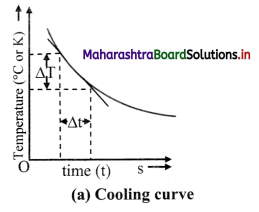6. Draw tangents to the curve at suitable points on the curve. The slope of each tangent is $$\lim _{\Delta t \rightarrow 0} \frac{\Delta \mathrm{T}}{\Delta \mathrm{t}}$$ and gives the rate of fall of temperature at that temperature (T).
7. Now the graph of $$\left|\frac{\mathrm{dT}}{\mathrm{dt}}\right|$$ on Y-axis against (T – T0) on X-axis is plotted with (0, 0) origin. The graph is straight line and passes through origin as shown in figure (b), which verities Newton’s law of cooling.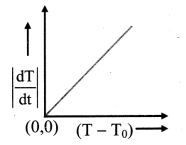(b) Graphical verification of Newton’s law of cooling

Question 16.
What is thermal stress? Give an example of disadvantages of thermal stress in practical use?

1. Consider a metallic rod of length l0 fixed between two rigid supports at T °C.
If the temperature of rod is increased by ∆T, length of rod would become,
l = l0(1 + α∆T)
Where, α is the coefficient of linear expansion of material of the rod.
But the supports prevent expansion of rod. As a result, rod exerts stress on the supports. Such stress is termed as thermal stress.
2. Disadvantage: Thermal stress can lead to fracture or deformation in substance under certain conditions.
3. Railway tracks are made up of metals which expand upon heating. If no gap is kept between tracks, in hot weather, expansion of metal tracks may exert thermal stress on track. This may lead to bending of tracks which would be dangerous. Hence, railway track is not a continuous piece but is made up of segments separated by gaps.Question 17.
Which materials can be used as thermal insulators and why?

1. Substances such as glass, wood, rubber, plastic, etc. can be used as thermal insulators.
2. These substances do not have free electrons to conduct heat freely throughout the body. Hence, they arc poor conductors of heat.

3. Solve the following problems.

Question 1.
A glass flask has volume 1 × 10-4 m3. It is filled with a liquid at 30 ºC. If the temperature of the system is raised to 100 ºC, how much of the liquid will overflow. (Coefficient of volume expansion of glass is 1.2 × 10-5 (ºC)-1 while that of the liquid is 75 × 10-5 ºC-1)
Solution:
Given: V1 = 1 × 10-4 m3 = 10-4 m3, T1 = 30°C,
T2 = 100 °C
To find: Volume of liquid that overflows
Formula: γ = $$\frac{V_{2}-V_{1}}{V_{1}\left(T_{2}-T_{1}\right)}$$
Calculation: From formula,
Increase is volume = V2 – V1
= γV1(T2 – T1)
increase in volume of beaker
= γglass × V1 (T2 – T1)
= 1.2 × 10-5 × 10-4 × (100 – 30)
= 1.2 × 70 × 10-9
= 4 × 10-9 m3
∴ Increase in volume of beaker
= 84 × 10-9 m3
Increase in volume of liquid
= γliquid × V1 (T2 – T1)
= 75 × 10-5 × 10 × (100 – 30)
= 75 × 70 × 10
= 5250 × 10-9 m3
∴ Increase in volume of liquid = 5250 × 10-9 m3
∴ Volume of liquid which overflows
= (5250 – 84) × 10-9 m3
= 5166 × 10-9 m3
= 0.5166 × 10-7 m3
Volume of liquid that overflows is 0.5166 × 10-7 m3.
[Note: The answer given above is presented considering standard conventions of writing number with its correct order of magnitude.]

Question 2.
Which will require more energy, heating a 2.0 kg block of lead by 30 K or heating a 4.0 kg block of copper by 5 K? (slead = 128 J kg-1 K-1, scopper = 387 J kg-1 K-1)
Solution:
mCu =4 kg, ∆TCu = 5 K,
sCu = 387 J/kg K
To find: Substance requiring more heat energy.
Formula: Q = ms ∆T
Calculation: From formula,
For Copper, QCu = 4 × 387 × 5 = 7740 J
QCu > Qlead, copper will require more heat energy.
Copper will require more heat energy.Question 3.
Specific latent heat of vaporization of water is 2.26 × 106 J/kg. Calculate the energy needed to change 5.0 g of water into steam at 100 ºC.
Solution:
Given: Lvap = 2.26 × 106 J/kg
m = 5g = 5 × 10-3 kg
In this case, no temperature change takes place only change of state occurs.
To find: Heat required to convert water into steam.
Formula: Heat required = mLvap
Calculation: From formula,
Heat required = 5 × 10-3 × 2.26 × 106
= 11300J
= 1.13 × 104 J
Heat required to convert water into steam is 1.13 × 104 J
[Note: The answer given above is presented considering standard conventions of writing number with its correct order of magnitude.]

Question 4.
A metal sphere cools at the rate of 0.05 ºC/s when its temperature is 70ºC and at the rate of 0.025 ºC/s when its temperature is 50 ºC. Determine the temperature of the surroundings and find the rate of cooling when the temperature of the metal sphere is 40 ºC.
Solution: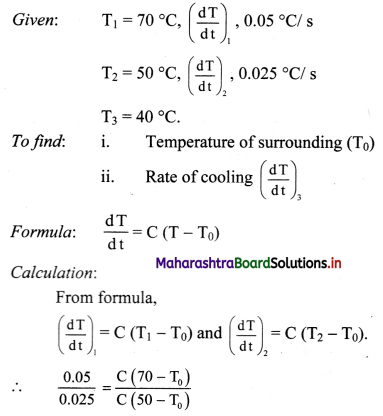∴ 2(50 – T0) = 70 – T0
∴ T0 = 30 πC
Substituting value of T0.
0.05 = C (70 – 30)
∴ C = $$\frac{0.05}{40}$$ = 0.00125/s.
For T3 = 40 °C
$$\left(\frac{\mathrm{d} \mathrm{T}}{\mathrm{dt}}\right)_{3}$$ = C(T3 – T0)
= 0.00125 (40 – 30)
= 0.00125 × 10
= 0.0125°C/s.
i) Temperature of surrounding is 30 °C.
ii) Rate of cooling at 40 °C is 0.0125 °C/s.

Question 5.
The volume of a gas varied linearly with absolute temperature if its pressure is held constant. Suppose the gas does not liquefy even at very low temperatures, at what temperature the volume of the gas will be ideally zero?
At temperature of -273.15 °C, the volume of the gas will be ideally zero.

Question 6.
In olden days, while laying the rails for trains, small gaps used to be left between the rail sections to allow for thermal expansion. Suppose the rails are laid at room temperature 27 ºC. If maximum temperature in the region is 45 ºC and the length of each rail section is 10 m, what should be the gap left given that α = 1.2 × 10-5 K-1 for the material of the rail section?
Solution:
Given. T1 = 27 °C, T2 = 45 °C,
L1 = 10m.
α = 1.2 × 10-5 K-1
To find: Gap that should be left (L2 – L1)
Formula: L2 – L1 = L1 α(T2 – T1)
Calculation: From formula,
L2 – L1 = 10 × 1.2 × 10-5 × (45 – 27)
= 2.16 × 10-3 m
= 2.16 mm
The gap that should be left between rail sections is 2.16 mm.

Question 7.
A blacksmith fixes iron ring on the rim of the wooden wheel of a bullock cart. The diameter of the wooden rim and the iron ring are 1.5 m and 1.47 m respectively at room temperature of 27 ºC. To what temperature the iron ring should be heated so that it can fit the rim of the wheel (αiron = 1.2 × 10-5 K-1).
Solution:
Given: dw = 1.5 m, d = 1.47 m, T1 = 27 °C.
αi = 1.2 × 10-5/ K
To find: Temperature (T2)
Formula. α = $$\frac{\mathrm{d}_{\mathrm{w}}-\mathrm{d}_{\mathrm{i}}}{\mathrm{d}_{\mathrm{i}}\left(\mathrm{T}_{2}-\mathrm{T}_{1}\right)}$$
Calculation: From formula,
T2 = $$\frac{\mathrm{d}_{\mathrm{w}}-\mathrm{d}_{\mathrm{i}}}{\mathrm{d}_{\mathrm{i}} \alpha}$$ + T1
= $$\frac{1.5-1.47}{1.47 \times 1.2 \times 10^{-5}}$$ + 27
= 1700.7 + 27
= 1727.7 °C
Iron ring should be heated to temperature of 1727.7 °C.Question 8.
In a random temperature scale X, water boils at 200 °X and freezes at 20 °X. Find the boiling point of a liquid in this scale if it boils at 62 °C.
Solution:
Here thermometric property P is temperature at some random scale X.
Using equation,
T = $$\frac{100\left(P_{T}-P_{1}\right)}{\left(P_{2}-P_{1}\right)}$$
For P1 = 20 °X,
P2 = 200 °X,
T = 62°C
∴ 62 = $$\frac{100\left(\mathrm{P}_{\mathrm{T}}-20\right)}{(200-20)}$$
∴ PT = $$\frac{62 \times(200-20)}{100}$$ + 20 = 111.6 + 20
= 131.6 °X
The boiling point of a liquid in this scale is 131.6 °X.

Question 9.
A gas at 900°C is cooled until both its pressure and volume are halved. Calculate its final temperature.
Solution:
Given: T1 = 900 °C = 900 + 273.15 = 1173.15 K
V2 = $$\frac{\mathrm{V}_{1}}{2}$$, P2 = $$\frac{\mathrm{P}_{1}}{2}$$
To find: Final temperature (T2)
Formula: $$\frac{\mathrm{P}_{1} \mathrm{~V}_{1}}{\mathrm{~T}_{\mathrm{I}}}=\frac{\mathrm{P}_{2} \mathrm{~V}_{2}}{\mathrm{~T}_{2}}$$
Calculation: From formula.
$$\frac{\mathrm{P}_{1} \mathrm{~V}_{1}}{1173.15}=\frac{\mathrm{P}_{1} \mathrm{~V}_{\mathrm{l}}}{4 \mathrm{~T}_{2}}$$
∴ T2 = $$\frac{1173.15}{4}$$ = 293.29 K
Final temperature of gas is 293.29 K.

Question 10.
An aluminium rod and iron rod show 1.5 m difference in their lengths when heated at all temperature. What are their lengths at 0 °C if coefficient of linear expansion for aluminium is 24.5 × 10-6 /°C and for iron is 11.9 × 10-6 /°C
Solution:
Given: (LT)i – (LT)al = 1.5 m, T0 = 0 °C
αal = 24.5 × 10-6/°C
αi = 11.9 × 10-6 /°C
To find: Lengths of aluminium and iron rod (L0)al and (L0)i
Formula: LT = L0[(1 + α(T – T0)]
Calculation: For T0 = 0 °C
From formula,
LT = L0(1 + αT)
For aluminium,
(L0)al = (L0)al(1 + αalT) ……………. (1)
For iron,
(LT)i = (L0)i (1 + αiT) ………….. (2)
Subtracting equation (2) by (1),
(LT)i – (LT)al = [(L0)i + (L0)i αiT] – [(L0)al + (L0)alαalT]
= (L0)i – (L0)al + [(L0)i αi – (L0)al αal]T
∴ 1.5 = 1.5 + [(L0)i αi – (L0)al αal)]T
⇒ [(L0)iαi – (L0)alαal] T = 0
∴ (L0)alαal = (L0)iαi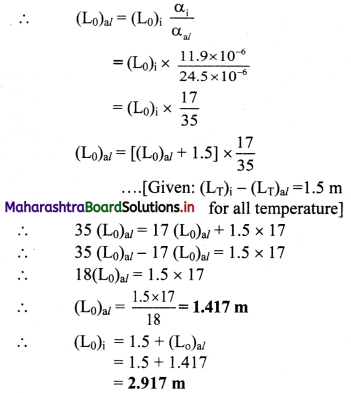Length of aluminium rod at 0 °C is 1.417 m and that of iron rod is 2.917 m.

Question 11.
What is the specific heat of a metal if 50 cal of heat is needed to raise 6 kg of the metal from 20°C to 62 °C ?
Solution:
Given: Q = 50 cal, m =6 kg,
∆T = 62 – 20 = 42 °C
To find: Specific heat (s)
Formula: Q = ms ∆T
Calculation: From formula,
s = $$\frac{\mathrm{Q}}{\mathrm{m} \Delta \mathrm{T}}=\frac{50}{6 \times 42}$$ = 0.198 cal/kg °C
Specific heat of metal is copper 0.198 cal/kg °C.Question 12.
The rate of flow of heat through a copper rod with temperature difference 30 °C is 1500 cal/s. Find the thermal resistance of copper rod.
Solution:
Given: ∆T = 30 °C, Pcond = 1500 cal/s
To find: Thermal resistance (RT)
Formula: RT = $$\frac{\Delta \mathrm{T}}{\mathrm{P}_{\text {cond }}}$$
Calculation: From formula,
RT = $$\frac{30}{1500}$$
= 0.02 °C s/cal.
Thermal resistance of copper rod is 0.02 °C s/cal.

Question 13.
An electric kettle takes 20 minutes to heat a certain quantity of water from 0°C to its boiling point. It requires 90 minutes to turn all the water at 100°C into steam. Find the latent heat of vaporisation. (Specific heat of water = 1cal/g°C)
Solution:
Let heat supplied by kettle in 20 minutes be Q1 and that in 90 min. be Q2.
Using heat temperature of water is raised from O °C to 100 °C.
If mass of water in the kettle is ‘m’ then.
Q1 = mswater∆T m × 1 × (100 – 0)
= 100 m ………….. (i)
…………. (∵ Swater = 1 cal/g °C)
Similarly using heat Q2 water is converted from liquid to gas,
∴ Q2 = mLvap ……………. (ii)
Given that heat Q1, Q2 are supplied to water in 20 min. (t1) and 90 min (t2) respectively.
Kettle being same its conduction rate (Pcond) is same.
Using Pcond = $$\frac{\mathrm{Q}_{1}}{\mathrm{t}_{1}}=\frac{\mathrm{Q}_{2}}{\mathrm{t}_{2}}$$ …………… (iii)
From (i), (ii) and (iii),
$$\frac{100 \mathrm{~m}}{20}=\frac{\mathrm{mL}_{\text {vap }}}{90}$$,
∴ Lvap = 5 × 90 = 450 cal/g
Latent heat of vaporisation for water is 450 cal/g.

Question 14.
Find the temperature difference between two sides of a steel plate 4 cm thick, when heat is transmitted through the plate at the rate of 400 k cal per minute per square metre at steady state. Thermal conductivity of steel is 0.026 kcal/m s K.
Solution: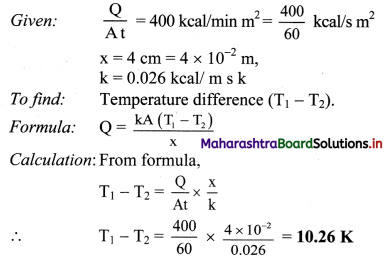Temperature difference between two sides is 10.26 K.
[Note: Above answer is expressed in K (‘kelvin considering that thermal conductivity is expressed in units of kcal / ms K, and not as kcal / m s °C. As 1 °C equivalent to 1 K. conceptually temperature difference of 10.26 K will correspond to 10.26 t]Question 15.
A metal sphere cools from 80 °C to 60 °C in 6 min. How much time with it take to cool from 60 °C to 40 °C if the room temperature is 30°C?
Solution:
Given: T1 = 80 °C, T2 = 60 °C, T3 = 40 °C, T0 = 30 °C, (dt)1 = 6 min.
To find: Time taken in cooling (dt)2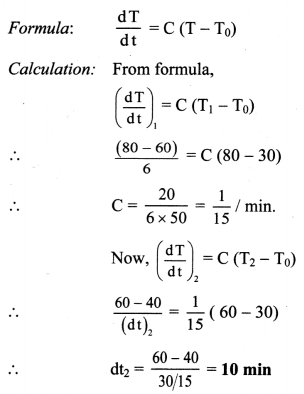Time taken in cooling is 10 min.

11th Physics Digest Chapter 7 Thermal Properties of Matter Intext Questions and Answers

Can you tell? (Textbook Page No. 125)

Question 1.
i) Why the metal wires for electrical transmission lines sag?
ii) Why a railway track is not a continuous piece but is made up of segments separated by gaps?
iii) How a steel wheel is mounted on an axle to fit exactly?

1. In hot weather, metal wires get heated due to increased temperature of surrounding. As a result, they expand increasing the slack between transmission line structure, causing them to sag.
2. Railway tracks are made up of metals which expand upon heating. If no gap is kept between tracks, in hot weather, expansion of metal tracks may exert thermal stress on track. This may lead to bending of tracks which would be dangerous. Hence, railway track is not a continuous piece but is made up of segments separated by gaps.
3. The steel wheel is heated to expand. This expanded wheel can easily fit over axle. The wheel is then cooled quickly. Upon cooling, wheel contracts and fits tightly upon the axle.

Intext question. (Textbook Page No 124)

Question 1.
Can you now tell why the balloon bursts sometimes when you try to fill air in it?

1. When balloon is blown, air that is blown inside makes the balloon expand.
2. A given size of balloon can expand upto certain limit.
3. Once that limit is reached and air is still blown inside the balloon, balloon cannot expand further.
4. As a result, air causes additional pressure on inner surface of balloon.
5. Since, pressure inside balloon is now greater than pressure outside balloon, balloon bursts equalizing the two pressures.Can you tell? (Textbook Page No. 125)

Question 1.
Why lakes freeze first at the surface?

1. In cold climate, temperature of water in ponds and lakes starts falling.
2. On getting colder, water contracts. As a result, density of water increases and it goes down. To replace it, warmer water from below rises up. This process continues till temperature of water at the bottom of pond becomes 4 °C.
3. Water, due to its anomalous behaviour possesses maximum density at 4 °C.
4. If the temperature lowers further, ice is formed at the surface of pond with water below it.
5. Ice being poor conductor of heat blocks the further heat exchange between atmosphere and water in the pond and maintains water below surface in liquid state.

Activity (Textbook Page No. 129)

Question 1.
To understand the process of change of state:
Take some cubes of ice in a beaker. Note the temperature of ice (0 °C). Start heating it slowly on a constant heat source. Note the temperature after every minute. Continuously stir the mixture of water and ice. Observe the change in temperature. Continue heating even after the whole of ice gets converted into water. Observe the change in temperature as before till vapours start coming out. Plot the graph of temperature (along Y-axis) versus time (along X-axis). Obtain a graph of temperature versus time.
[Students are expected to attempt the activity on their own.]

Can you tell? (Textbook Page No. 130)

Question 1.
What is observed after point D in graph? Can steam be hotter than 100 °C?
Beyond point D, thermometer again shows rise in temperature. This means, steam can be hotter than 100 °C and is termed as superheated steam.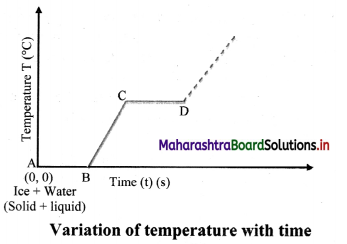Question 2.
Why steam at 100 °C causes more harm to our skin than water at 100 °C?

1. Though steam and boiling water have same temperature, the heat contained in steam is more than that in boiling water.
2. Steam is formed when boiling water absorbs specific latent heat of vaporisation i.e.. 22.6 × 105 J/kg.
3. As a result, when steam comes in contact with the skin of a person, it gives off additional 22.6 × 105 joule per kilogram causing severe (more serious) burns.
Hence, burns caused from steam are more serious than those caused from boiling water at same temperature.

Activity (Textbook Page No. 130)

Activity to understand the dependence of boiling point on pressure:
Take a round bottom flask, more than half filled with water. Keep it over a burner and fix a thermometer and steam outlet through the cork of the flask as shown in figure. As water in the flask gets heated, note that first the air, which was dissolved in the water comes out as small bubbles. Later bubbles of steam form at the bottom but as they rise to the cooler water near the top, they condense and disappear. Finally, as the temperature of the entire mass of the water reaches 100 oc, bubbles of steam reach the surface and boiling is said to occur. The steam in the flask may not be visible hut as it comes out of the flask, It condenses as tiny droplets of water giving a foggy appearance.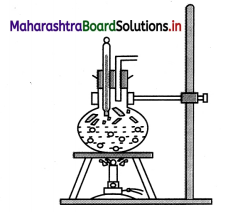If now the steam outlet is closed for a few seconds to increase the pressure in the flask, you will notice that boiling stops. More heat would be required to raise the temperature (depending on the increase in pressure) before boiling starts again. Thus, boiling point increases with increase in pressure. Let us now remove the burner. Allow water to cool to about 80°C. Remove the thermometers and steam outlet. Close the flask with a air tight cork. Keep the flask turned upside down on a stand. Pour icecold water on the flask. Water vapours in the flask condense reducing the pressure on the water surface inside the flask. Water begins to boil again, now at a lower temperature. Thus boiling point decreases with decrease in pressure and increases with increase in pressure.
[Students are expected to attempt the activity an their own.]Can you tell? (Textbook Page No. 131)

Question 1.
i) Why is cooking difficult at high altitude?
ii) Why is cooking faster in pressure cooker?

• At high altitude density of air is low which causes reduction in atmospheric pressure.
• As pressure is less, boiling point of water lowers.
• Water, at high altitude, starts boiling below 100 OC.
• As food is cooked mostly through the water boiling, cooking of food becomes difficult.
• Pressure cooker operates by expelling air within the cooker and trapping steam produced from the liquid. (mostly water) boiling inside.
• Due to high internal pressure, boiling point of liquid increases and liquid boils at temperature higher than its boiling point.
• The increased boiling point allows more absorption of heat by liquid and steam formed is superheated.
• As a result, food gets cooked quickly.

Internet my friend (Textbook Page No. 139)

i) https ://hyperphysics. phy-astr.gsu.edul/base/hframe.html
ii) https://youtu.be/7ZKHc5J6R5Q
iii) https://physics. info/expansion